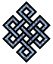#fail2ban bad ip database: ip 139.199.181.192

### | ip database | live view | stats | report | help | api key:

 ip: 139.199.181.192 hostname: 139.199.181.192 country:[CN] China first reported: 05.10.2018 21:36.33 GMT+0200 last reported: 16.01.2019 03:34.41 GMT+0200 time period: 102d 06h 58m 08s total reports: 10 reported by: 3 host(s) filter(s): ssh (6) ssh (4) tor exit node no badips.com db Lookup## port scan of '139.199.181.192':

[-hide]
```# Nmap 6.40 scan initiated Fri Oct  5 21:37:03 2018 as: /usr/bin/nmap -sU -sS -O 139.199.181.192
Warning: 139.199.181.192 giving up on port because retransmission cap hit (10).
Nmap scan report for 139.199.181.192
Host is up (0.30s latency).
Not shown: 1873 closed ports, 89 open|filtered ports, 37 filtered ports
PORT   STATE SERVICE
22/tcp open  ssh
No exact OS matches for host (If you know what OS is running on it, see http://nmap.org/submit/ ).
TCP/IP fingerprint:
OS:SCAN(V=6.40%E=4%D=10/5%OT=22%CT=1%CU=2%PV=N%G=Y%TM=5BB7D87B%P=x86_64-pc-
OS:linux-gnu)SEQ(TS=A)SEQ(CI=RD%TS=E)ECN(R=Y%DF=Y%TG=40%W=7210%O=M590NNSNW7
OS:%CC=Y%Q=)T1(R=Y%DF=Y%TG=40%S=O%A=S+%F=AS%RD=0%Q=)T2(R=N)T3(R=N)T4(R=Y%DF
OS:=Y%TG=40%W=0%S=A%A=Z%F=R%O=%RD=0%Q=)T5(R=Y%DF=Y%TG=40%W=0%S=Z%A=S+%F=AR%
OS:O=%RD=0%Q=)T6(R=Y%DF=Y%TG=40%W=0%S=A%A=Z%F=R%O=%RD=0%Q=)T7(R=Y%DF=Y%TG=4
OS:0%W=0%S=Z%A=S+%F=AR%O=%RD=0%Q=)U1(R=N)IE(R=N)

OS detection performed. Please report any incorrect results at http://nmap.org/submit/ .
# Nmap done at Fri Oct  5 23:32:43 2018 -- 1 IP address (1 host up) scanned in 6941.25 seconds
```
```Σ = 51 | Δt = 0.0042018890380859s
```